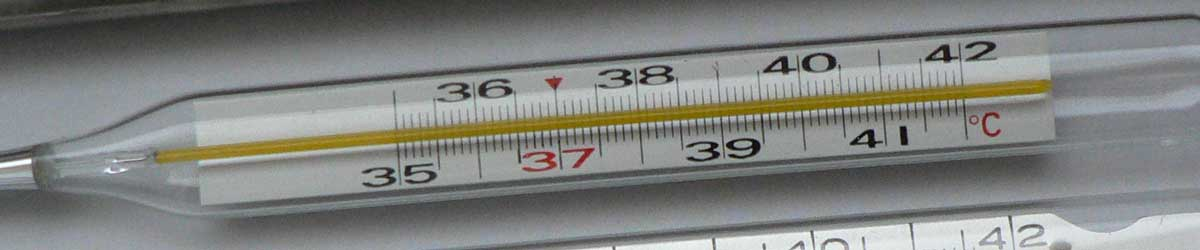# Delisle to Rankine Conversion

Enter the temperature in Delisle below to get the value converted to Rankine.

Results in Degrees Rankine:1 °De = 670.47 °R
Do you want to convert Rankine to Delisle?

## How to Convert Delisle to RankineConvert degrees Delisle to degrees Rankine with this simple formula:

degrees Rankine = 671.67 - [°De] × 6 / 5

Insert the [°De] temperature measurement in the formula and then solve to find the result.

For example, let's convert 50 °De to degrees Rankine:

50 °De = ( 671.67 - 50 × 6 / 5 ) = 611.67 °R

## Delisle

The Delisle scale is a temperature scale that sets a fixed zero point equal to the temperature of boiling water. The Delisle scale is uses incrementing degrees as the temperature decreases. It runs from 0 degrees at the boiling point of water to 150 degrees at the the freezing point.

Delisle can be abbreviated as De; for example, 1 degree Delisle can be written as 1 °De.

### Important Temperatures In Degrees Delisle

TemperatureDegrees Delisle
Absolute Zero559.725 °De
Freezing Point of Water150 °De
Boiling Point of Water0 °De

## Rankine

The Rankine scale is an absolute temperature scale that uses absolute zero as the starting point, much like the Kelvin scale. The key difference between the Rankine scale and the Kelvin scale is that an increment of one degree rankine is equal to an increment of one degree Fahrenheit, not Celsius as in the Kelvin scale. The base of the Rankine scale starts at absolute zero, thus 0 degrees rankine are equal to −459.67 degrees Fahrenheit.

Rankine can be abbreviated as R, and are also sometimes abbreviated as Ra. For example, 1 degree Rankine can be written as 1 °R or 1 Ra.

### Important Temperatures In Degrees Rankine

TemperatureDegrees Rankine
Absolute Zero0 °R
Freezing Point of Water491.67 °R
Triple Point of Water491.688 °R
Boiling Point of Water671.67 °R

## Delisle to Rankine Conversion Table

Delisle measurements converted to Rankine
Delisle Rankine
-50 °De 731.67 °R
-40 °De 719.67 °R
-30 °De 707.67 °R
-20 °De 695.67 °R
-10 °De 683.67 °R
0 °De 671.67 °R
10 °De 659.67 °R
20 °De 647.67 °R
30 °De 635.67 °R
40 °De 623.67 °R
50 °De 611.67 °R
60 °De 599.67 °R
70 °De 587.67 °R
80 °De 575.67 °R
90 °De 563.67 °R
100 °De 551.67 °R
110 °De 539.67 °R
120 °De 527.67 °R
130 °De 515.67 °R
140 °De 503.67 °R
150 °De 491.67 °R
160 °De 479.67 °R
170 °De 467.67 °R
180 °De 455.67 °R
190 °De 443.67 °R
200 °De 431.67 °R
300 °De 311.67 °R
400 °De 191.67 °R
500 °De 71.67 °R
559.73 °De 0 °R
600 °De -48.33 °R
700 °De -168.33 °R
800 °De -288.33 °R
900 °De -408.33 °R
1,000 °De -528.33 °R